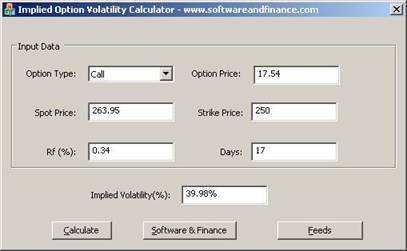# Implied Option Volatility Calculator

 Implied Option Volatility CBOE volatility Index Steps For Calculating Implied Option Volatility C++ Program For Implied Option Volatility Input Data Output Data Download

Implied Option Volatility

Implied volatility of a stock is the future volatility and is calculated using the derivatives of the underlying stock. The factors used in calculating implied volatility are given below:

1. Risk Free Rate
2. strike price
3. Spot price
4. time to maturity
5. option's price.

Top

CBOE volatility Index

The ticker symbol for CBOE volatility index is VIX. It represents the implied options volatility of S&P 500. It includes the out of the money options also for calculation. VXO is another ticker symbol and it does include only at the money options.

Steps For Calculating Implied Options Volatility

1. Get the last traded call or put option price, strike price, spot price

2. Get the Risk Free Rate - Rf and Time to maturity in number of day

3. Given the stock historical volatility, finding the option price is easier using black–scholes formula. To know more Black–Scholes Formula, click here.

4. But finding the volatility using this formula is a bit difficult and we need to use iterative method. However I am finding implied volatility using bisection method by taking a higher number as volatility for upper range and 0 as lower range. Get the option price using black–scholes formula.

5. If the calculated option price is higher than the given option price, then volatility of upper range needs to go down to (lower + upper)/2.

6. If the calculated option price is lower than the given option price, then volatility of lower range needs to go up to (lower + upper)/2.

7. When the calculated option price is equal to given option price with two decimal point accuracy, the algorithm exits the correct implied volatility.

Sample Program For Calculating Implied Option Volatility

Here is the C++ program to calculate Implied Option Volatility.

double COptionCalculator::GetImpliedOptionVolatilityForCallOption(double strike, double spot, double days, double callOptionPrice, double Rf)

{

double cpTest = 0;

double IV = 500;

double upper = 500;

double lower = 0;

double range = fabs(lower - upper);

bool dnword = true;

while(1)

{

// Get the call option price using black scholes formula
// The code GetCallOptionPrice using black–scholes formula
// is not given

cpTest = GetCallOptionPrice(strike, spot, days, IV, Rf);

if(cpTest > callOptionPrice)

{  // Implied Volatility - IV  has to go down

upper = IV;

IV = (lower + upper) / 2;

}

else

{

// Implied Volatility - IV has to go up

lower = IV;

IV = (lower + upper) / 2;

}

range = fabs(lower - upper);

if( range < 0.001)

break;

}

return IV;

}

Top

Input Data

There are 6 inputs shown below

1. Call or Put option
2. Risk Free Rate
3. strike price
4. Spot price
5. time to maturity
6. option's price.

Top

Output Data

Refer to the attached screen shotYou can cross check the results using CBOE volatility free service.
Top